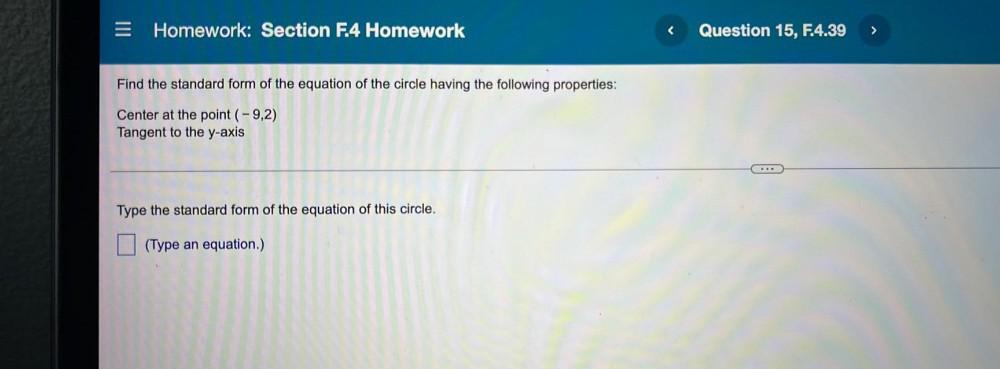Question:

# = Homework: Section F.4 Homework Question 15, F.4.39 > Find the standard form of the equation of the circle having the following= Homework: Section F.4 Homework Question 15, F.4.39 > Find the standard form of the equation of the circle having the following properties: Center at the point (-9,2) Tangent to the y-axis Type the standard form of the equation of this circle. (Type an equation.)